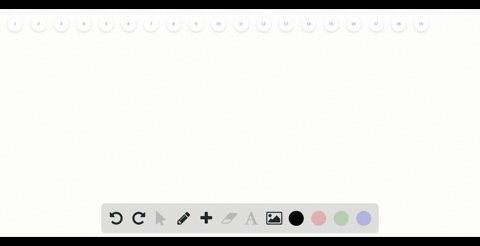Sign up for our free STEM online summer camps starting June 1st!View Summer Courses### Determine the empirical and molecular formulas of…

09:09Auburn University Main Campus

Need more help? Fill out this quick form to get professional live tutoring.

Get live tutoring
Problem 52

What is the molecular formula of each of the following compounds?
$$\begin{array}{l}{\text { (a) empirical formula } \mathrm{HCO}_{2} \text { , molar mass }=90.0 \mathrm{g} / \mathrm{mol}} \\ {\text { (b) empirical formula } \mathrm{C}_{2} \mathrm{H}_{4} \mathrm{O}, \text { molar mass }=88.0 \mathrm{g} / \mathrm{mol}}\end{array}$$

a) See explanation for solution.
b) See explanation for solution.

## Discussion

You must be signed in to discuss.

## Video Transcript

Okay, Sister, Be the first one here and we ah consider the molecule of formal off then pickle formula. It's your two. As molecular formula is equal to h See, orto your record n So it's suit with the empirical formal and we have to find out the value ofthe end to find this. Find the molecular formula so that in here is the the mole armas off the compound over them. Empirical formula mus So here it is, the more enormous or the or the molecular muss over them Miracle formula mus so we can find them Brickell formula mus from here just adding up, um, the formula mus for each adorable space is present here some the empirical formula mus for its your two will be for one hydrogen we have here and the hydra sins and bagel or the prisons for mama says one point zero zero eight and we only want had risen. Plus, we have one carbon and Carmen's former Thomas's to have a mind zero one and we have two oxygen oxygen from one of us is sixteen and we have through oxygen. That means we'LL have two multiplied by two and this someone give us, um, forty five point zero two and this is a unit off Grandpa Ramon. So here is the empirical form Lamas for its Soto and were given the mole Armas as ninety grand Permal. So we just put these values here Similar mass of these components Given us nineteen, ninety grand Permal and empirical formulas, we have calculated as forty five point zero two Grandpa Ramon, and this will give us the value ofthe n as one point nine nine. And we can assume this as too, since we need a whole number or enter your number or the well off end. So now we can just place the Vela off in here to find a molecule of formula. So the molecule of formal will be each C or two whole. Break it, say that there is too. And we just multiplication by tools which will be each too C two, four, four. So this is the molecular formula for them pickle formal and the molecular must given now for the second one the same way we have them breaking formula situation for or so the molecular formula will be sideways for well, break it in and find in again is the Moler Mayes over the empirical formal a month. All right, Now we know the molar must given as eighty eight gram per mall, you need to find out the empirical form. All of us. Was she confined from this formula? So then Brickell formula mus for C two h for all with a name. So you have to to carbon will have twelve point zero one times two class. We have four had risen net men's We have one point zero zero eight times four plus we have one oxygen sixteen and the total will give us a fellow forty four point zero five. Grandpa are more now. Just put this value here. Forty four point zero five. Grandpa Ramon, this will give us again. This only visible off approximately one point nine nine or approximately to so just put this value here to get the molecular formula So the molecular formula will be see to age for or more break it too. Give us C four h eight Oh two. So here is the molecular formula for the empirical formula off this one and the Moler mus off eighty eight gram